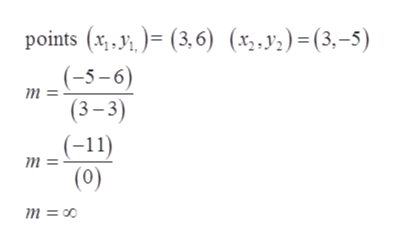# What would be the linear equation for this function, & the graph??(X1,Y1)=(3,6), (X2,Y2)=(3,-5)

Question

What would be the linear equation for this function, & the graph??

(X1,Y1)=(3,6), (X2,Y2)=(3,-5)

check_circleExpert Solution
Step 1

What would be the linear equation for this function, & the graph??

(X1, Y1) = (3,6), (X2, Y2)=(3,-5)

Given:

Step 2

Formula used:

Step 3

To find the slope ...help_outlineImage Transcriptioncloseрoints (x.У,)- (3,6) (х.5.) - (3.-5) (-5-6) т (3-3) (-11) (0) т т %3 00 fullscreen

### Want to see the full answer?

See Solution

#### Want to see this answer and more?

Solutions are written by subject experts who are available 24/7. Questions are typically answered within 1 hour*

See Solution
*Response times may vary by subject and question
Tagged in

### Applications of Mathematics